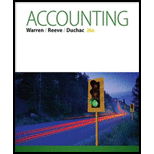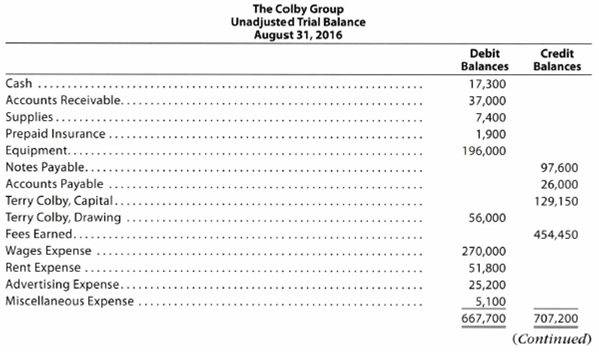Chapter 2, Problem 2.5APR### Accounting (Text Only)

26th Edition
Carl Warren + 2 others
ISBN: 9781285743615

#### Solutions

Chapter
Section### Accounting (Text Only)

26th Edition
Carl Warren + 2 others
ISBN: 9781285743615
Textbook Problem
24 views

# Corrected trial balanceThe Colby Group has the following unadjusted trial balance as of August 31, 2016:The debit and credit totals are not equal as a result of the following errors: a. The cash entered on the trial balance was understated by $6,000. b. A cash receipt of$5,600 was posted as a debit to Cash of $6,500. c. A debit of$11,000 to Accounts Receivable was not posted. d. A return of $150 of defective supplies was erroneously posted as a$1,500 credit to Supplies. e. An insurance policy acquired at a cost of $1, 200 was posted as a credit to Prepaid Insurance. f. The balance of Notes Payable was understated by$20,000. g. A credit of $4,800 in Accounts Payable was overlooked when determining the balance of the account. h. A debit of$7,000 for a withdrawal by the owner was posted as a credit to Terry Colby, Capital. i. The balance of $58, 100 in Rent Expense was entered as$51,800 in the trial balance. j. Gas, Electricity, and Water Expense, with a balance of $24,150, was omitted from the trial balance. Instructions 1. Prepare a corrected unadjusted trial balance as of August 31, 2016. 2. Does the fact that the unadjusted trial balance in (1) is balanced mean that there are no errors in the accounts? Explain. 1. To determine Corrected unadjusted trial balance: The corrected unadjusted trial balance is the summary of all the ledger accounts that appears on the ledger accounts after making adjusting journal entries. The corrected unadjusted trial balance is also known as adjusted trial balance. To prepare: A corrected unadjusted trial balance as of August 31, 2016. Explanation Working notes: a. Cash={(Cash (unadjusted)+Understated cashIncorrect posting of cash receipt debited to cash account (b.))}={($17300+$6,000)($6,500$5,600)}=$22,400 (1)

c.

An amount of $11,000 was not debited to accounts receivable (asset) account. Hence, by debiting the amount, the accounts receivable in the corrected unadjusted trial balance would be$48,000[$37,000(unadjusted)+$11,000].

d.

As a return of defective supplies of $150 was wrongly credited to the supplies (asset) account as$1,500, it is to be corrected by debiting the Supplies account with an amount of $1,350($1,500$150). Hence, the amount of Supplies in the corrected unadjusted trial balance would be$8,750[$7,400(unadjusted)+$1,350].

e.

As the purchase of insurance policy at a cost of $1,200 was wrongly credited to prepaid insurance (asset) account, it is to be corrected by crediting the Prepaid insurance account with an amount of$1,200. Hence, the amount of Prepaid insurance in the corrected unadjusted trial balance would be $3,100[$1,900(unadjusted)+\$1,200].

f

2.

To determine

To Discuss: Whether the fact of the balancing of unadjusted trial balance in (1) means that there are no errors in the accounts.

### Still sussing out bartleby?

Check out a sample textbook solution.

See a sample solution

#### The Solution to Your Study Problems

Bartleby provides explanations to thousands of textbook problems written by our experts, many with advanced degrees!

Get Started

#### Find more solutions based on key concepts